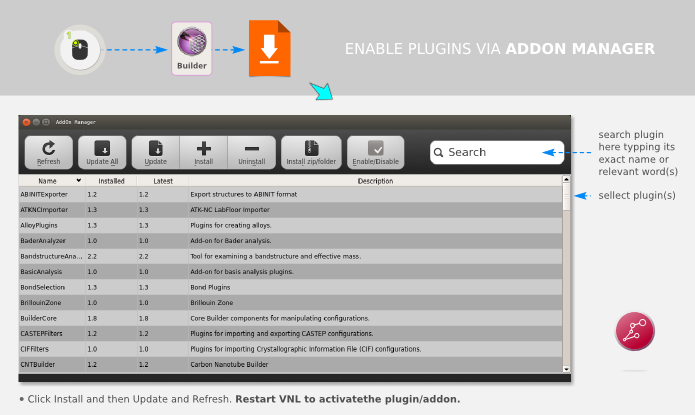# Converting lattices: Rhombohedral to hexagonal and back¶

Version: 2015.1

In this tutorial, you will learn how to convert hexagonal (hP) Bravais lattices to rhombohedral (hR) ones and vice versa. Both of these lattices belong to the Trigonal crystal system.As we know, there are 14 Bravais lattices in $$\mathbf{R}^3$$ space. These determine the translational symmetry properties of a crystal unit cell, and thereby also the symmetry properties in reciprocal space (high-symmetry k-points, etc.). Additionally, the space group determines the rotational and other internal symmetry operations of the basis (the atomic coordinates). For the complete symmetry specification we need both, although the lattice symmetry is usually determined by the space group, i.e. each space group is associated with one and only one Bravais lattice.

However, the trigonal crystal system is special, because one can often choose between rhombohedral and hexagonal representation. Trigonal structures found in online databases are often hexagonal, but for computational purposes, the smaller rhombohedral cell will usually be more efficient. In that case, conversion between the two representations is adviseable.

Note

hR (hexagonal) and hP (rhombohedral) crystal systems

The space groups are classified by crystal systems, and any standard book on solid state physics will tell you that there are 7 crystal systems and 6 crystal families. Here we focus on the special situation in the hexagonal family, which is the only one that can be subdivided into two crystal systems; the hexagonal and the trigonal.

The hexagonal system comprises 27 space groups. All of these have hexagonal Bravais lattices, labeled hP. The trigonal system is the tricky one, because its 25 space groups (143-167) belong either to the hexagonal (hP, 18 space groups) or the rhombohedral (hR, 7 space groups) Bravais lattice. Now, to make things a bit more confusing, all trigonal crystals with rhombohedral lattices (space groups 146, 148, 155, 160, 161, 166, and 167), can be represented as an equivalent hexagonal system; there is a choice of using a hexagonal or a rhombohedral representation.

Further information on this subject is available in the section Crystal classifications.

## Conversion between hP and hR representations¶The hexagonal setting is in fact a supercell with three irreducible rhombohedral units. In the modern so-called obverse representation, the origins of these three subsystems are placed at the $$\boldsymbol{d}_1,\boldsymbol{d}_2,\boldsymbol{d}_3$$ fractional coordinates of the hexagonal lattice.

Tip

• $$\{\boldsymbol{A}_H,\boldsymbol{B}_H,\boldsymbol{C}_H\}_{hP}$$ : hexagonal (hP) lattice vectors
• $$\{\boldsymbol{A}_R,\boldsymbol{B}_R,\boldsymbol{C}_R\}_{hR}$$ : rhombohedral (hR) lattice vectors.

Taking into account the symmetries of the crystal structures we can find the following relations among the above vectors:

$\begin{split}\begin{pmatrix} \boldsymbol{d}_1\\ \boldsymbol{d}_2\\ \boldsymbol{d}_3 \end{pmatrix}= \frac{1}{3} \begin{pmatrix} 0 & 0 & 0 \\ 2 & 1 & 1 \\ 1 & 2 & 2 \end{pmatrix} \begin{pmatrix} \boldsymbol{A}_H\\ \boldsymbol{B}_H\\ \boldsymbol{C}_H \end{pmatrix}= \begin{pmatrix} 0 & 0 & 0 \\ 0 & 1 & 0 \\ 1 & 1 & 0 \end{pmatrix} \begin{pmatrix} \boldsymbol{A}_R\\ \boldsymbol{B}_R\\ \boldsymbol{C}_R \end{pmatrix}\end{split}$

The lattice vectors are connected via the following transformation (direct and inverse):

$\begin{split}\begin{pmatrix} \boldsymbol{A}_R\\ \boldsymbol{B}_R\\ \boldsymbol{C}_R \end{pmatrix} = \underbrace{ \frac{1}{3} \begin{pmatrix} -1 & 1 & 1 \\ 2 & 1 & 1 \\ -1 & -2 & 1 \end{pmatrix} }_{:=\boldsymbol{M}} \begin{pmatrix} \boldsymbol{A}_H\\ \boldsymbol{B}_H\\ \boldsymbol{C}_H \end{pmatrix} \Leftrightarrow \begin{pmatrix} \boldsymbol{A}_H\\ \boldsymbol{B}_H\\ \boldsymbol{C}_H \end{pmatrix} = \underbrace{ \begin{pmatrix} -1 & 1 & 0 \\ 1 & 0 & -1 \\ 1 & 1 & 1 \end{pmatrix} }_{:=\boldsymbol{M}^{-1}} \begin{pmatrix} \boldsymbol{A}_R\\ \boldsymbol{B}_R\\ \boldsymbol{C}_R \end{pmatrix}\end{split}$

Choosing now Cartesian coordinates one obtains the following relations:

$\begin{split}\textrm{hP:} \begin{cases} & \boldsymbol{A}_H = a_H(1,-\sqrt{3},0)/2 \\ & \boldsymbol{B}_H = a_H(1,+\sqrt{3},0)/2 \\ & \boldsymbol{C}_H = c_H(0,0,1) \end{cases}\hspace{1.cm},\hspace{.1cm}\textrm{hR:} \begin{cases} & \boldsymbol{A}_R = (0,+a_H\sqrt{3},c_H)/3 \\ & \boldsymbol{B}_R = (3a_H,-a_H\sqrt{3},2c_H)/6 \\ & \boldsymbol{C}_R = (-3a_H,-a_H\sqrt{3},2c_H)/6 \end{cases}\end{split}$

Warning

The orientation of the last cell with respect to the cartesian axes is different from the standard QuantumATK choice for the rhombohedral cell!

For later use, let us compute the lattice parameters of the rhombohedral cell, i.e. the lengths of the lattice vectors ($$a_H,c_H,a_R$$) and their angles ($$\alpha_R,\beta$$). They are all the same, as expected for a rhombohedral crystal, and after some algebra we obtain

$\begin{split}\begin{cases} & a_R=a_H\beta/3 \\ & \alpha_R=2\arcsin(3\beta/2)\\ & \textrm{where: }\beta:=\sqrt{3+(c_H/a_H)^2} \end{cases}\hspace{.1cm}\Rightarrow\hspace{.2cm} \begin{cases} & a_H=2a_R\sin(a_R/2) \\ & c_H=a_R3\sqrt{1+2\cos a_R} \end{cases}\end{split}$

Now, what to do in practice, if you have recevied a rhombohedral crystal in the hexagonal supercell representation, but want to convert it to the smaller rhombohedral system? We give the recipe in the next section.

## Converting hP supercell to hR primitive cell¶

Assume you have found a structure in some online database: Hilgardite, KS2Al3O14H6. Download the CIF file and save it: AMS_DATA.cif.

• Step 1: Drag and drop (or use Add ‣ From Files) the AMS_DATA.cif file on the Builder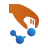.

• Step 2: You can read off the lattice parameters via Bulk Tools ‣ Lattice Parameters (or you can read them in the CIF file):

$\begin{split}& a_H = 6.96\textrm{ Angstroms} \\ & c_H = 17.35\textrm{ Angstroms}\end{split}$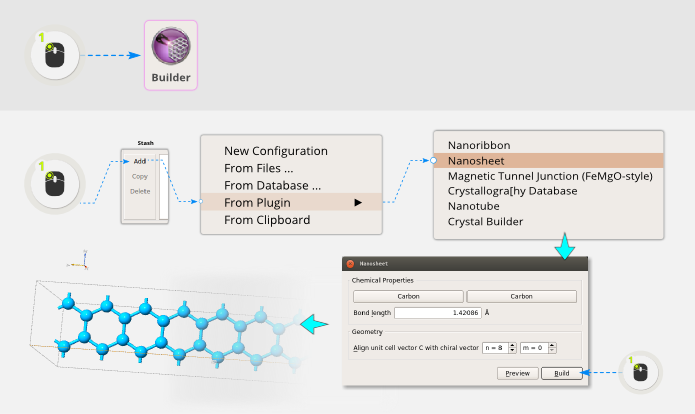• Step 3: To change the lattice to rhombohedral (hR), start by changing the “Lattice type” to “Unit cell”, and select to “Keep Cartesian coordinates constant when changing the lattice”. The rhombohedral primitive vectors are given by the relations from above and inserting the hexagonal lattice constants, you obtain:
$\begin{split}\textrm{hR:} \begin{cases} & \boldsymbol{A}_R = (0,+a_H\sqrt{3},c_H)/3 & = (0, 4.018, 5.7833)\\ & \boldsymbol{B}_R = (3a_H,-a_H\sqrt{3},2c_H)/6 & = (3.48, -2.009, 5.7833)\\ & \boldsymbol{C}_R = (-3a_H,-a_H\sqrt{3},2c_H)/6 & = (-3.48, -2.009, 5.7833) \end{cases}\end{split}$

Insert these numbers, component by component, in the Primitive Vectors table. (At this point you will see that the K atoms sit close to the corners of the unit cell, indicating that we have indeed made a reasonable transformation.)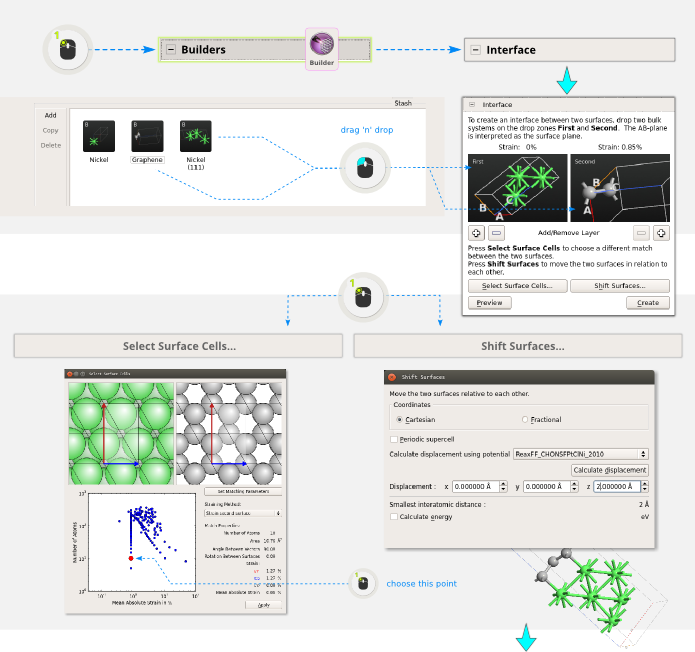• Step 4: As mentioned above, the orientation of this new cell with respect to the Cartesian axes is different from the standard QuantumATK choice for the rhombohedral cell. The next step is therefore to reorient the cell to fit the standard cell.
• To do this, switch to “Keep fractional coordinates constant when changing the lattice”. This ensures we do not distort the geometry while reorienting the cell.

• Change the “Lattice type” to “Rhombohedral”. This operation preserves the lengths of the primitive vectors, but aligns them into the standard orientation (and also uses the Rhombohedral lattice class, so you have access to the relevant symmetry points for band structure calculations, etc). As a verification of this, you can compute the lattice constants by hand:

$\begin{split}& \beta = 3.03548 \\ & a_R = a_H\beta/3 = 7.0423\textrm{ Angstroms}\\ & \alpha_R = 2\arcsin(3\beta/2) = 59.228^\circ\end{split}$

which is close to the values shown in the Lattice Parameters widget (some rounding error will occur due to the number of decimals used for the lattice vector components).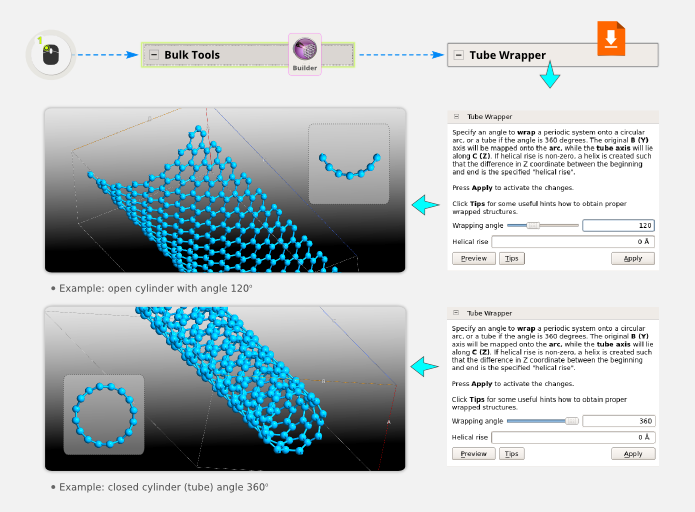• Step 5: There are still too many atoms (three times too many). These extra atoms are however all sitting in symmetry equivalent positions of the rhombohedral lattice, and thus by wrapping them into the cell, they will fall on top of the minimal basis. Thus, go to Bulk Tools ‣ Wrap, ensure all directions A, B, C are ticked, and click Apply.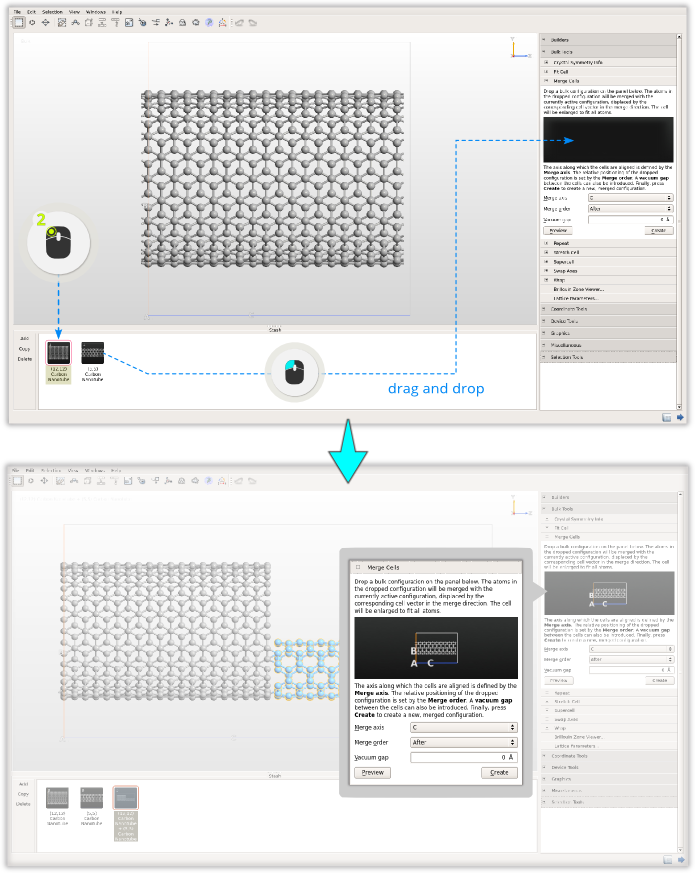• Step 6: Now it may appear as if magically the superfluous atoms were removed. However, they are still there - there are atoms on top of each other now. Such a configuration is not valid for calculations, so the final step is to remove the equivalent atoms. For this, use Select ‣ Close Neighbors, and click the buttons Select and Delete.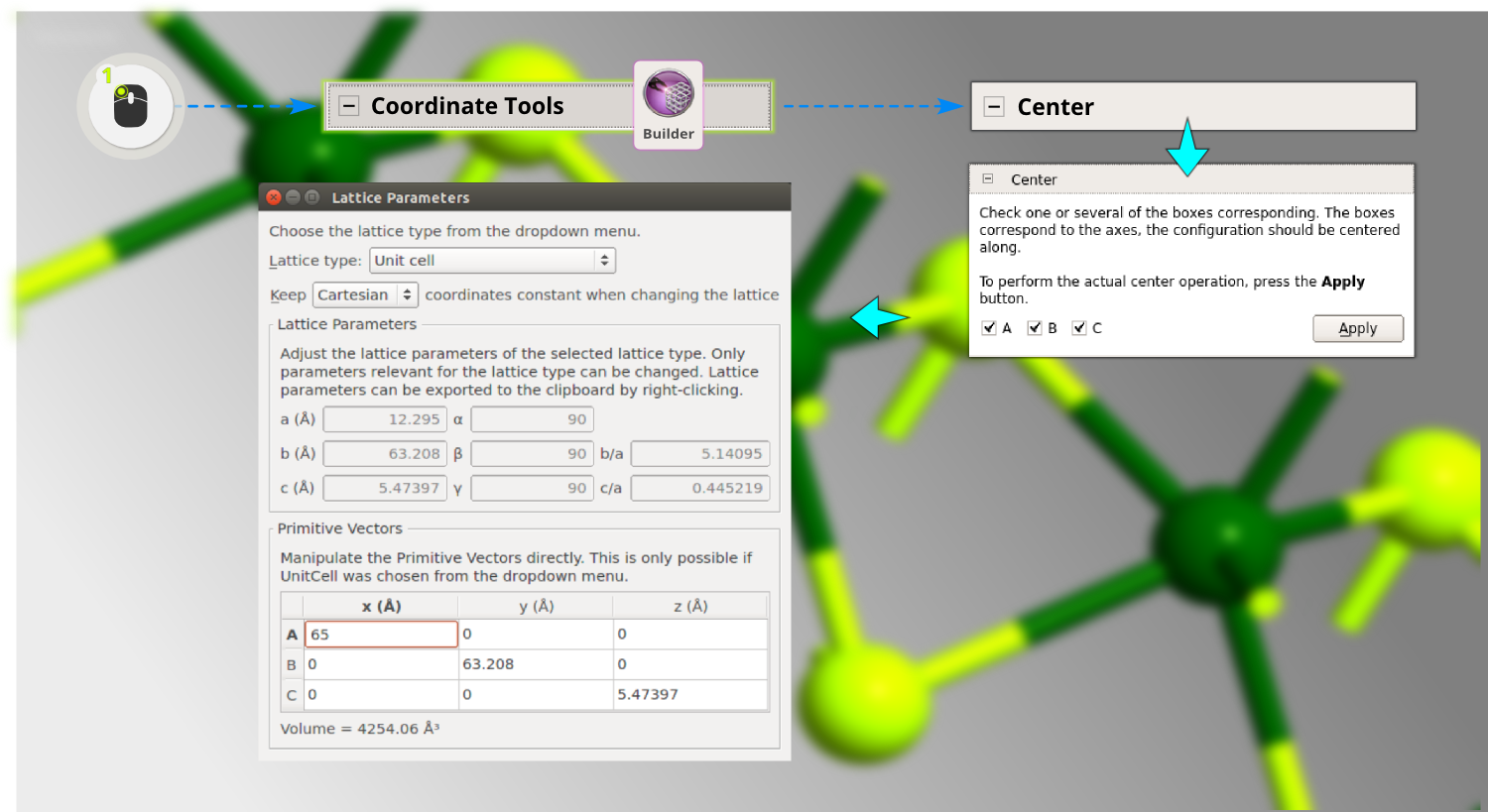Inverse case

You can of course also convert the other way, i.e. to the hexagonal supercell from the rhombohedral minimal cell. This is simpler. Looking above, we see that the hP vectors are obtained by hR after the action of the matrix $$\boldsymbol{M}^{-1}$$ as defined above. This matrix can be implemented directly in ATK by choosing Bulk Tools ‣ Supercell and enter this transformation:You now have a hexagonal cell, it’s just not oriented in the usual way, but this is easily fixed just like before: Use Tools ‣ Lattice Parameters, choose to keep fractional coordinates constant, then change the “Lattice type” to “Hexagonal”. The structure is now back to the originally imported file.

## Crystal classifications¶